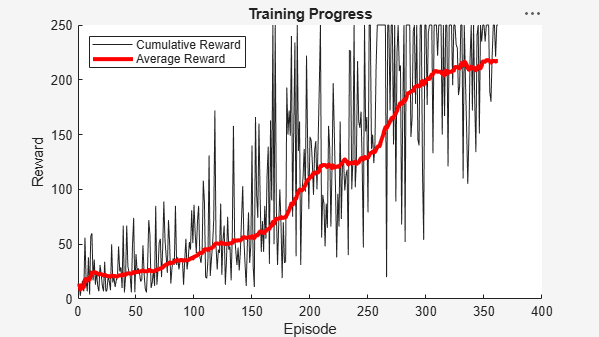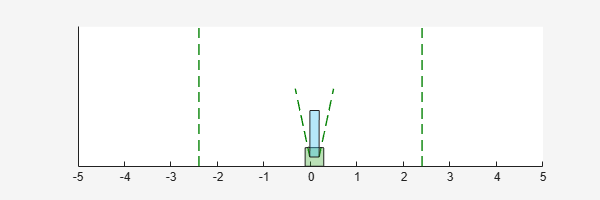Main Content

# Train Reinforcement Learning Policy Using Custom Training Loop

This example shows how to define a custom training loop for a reinforcement learning policy. You can use this workflow to train reinforcement learning policies with your own custom training algorithms rather than using one of the built-in agents from the Reinforcement Learning Toolbox™ software.

Using this workflow, you can train policies that use any of the following policy and value function representations.

In this example, a stochastic actor policy with a discrete action space is trained using the REINFORCE algorithm (with no baseline). For more information on the REINFORCE algorithm, see Policy Gradient Agents.

Fix the random generator seed for reproducibility.

`rng(0)`

For more information on the functions you can use for custom training, see Functions for Custom Training.

### Environment

For this example, a reinforcement learning policy is trained in a discrete cart-pole environment. The objective in this environment is to balance the pole by applying forces (actions) on the cart. Create the environment using the `rlPredefinedEnv` function.

`env = rlPredefinedEnv('CartPole-Discrete');`

Extract the observation and action specifications from the environment.

```obsInfo = getObservationInfo(env); actInfo = getActionInfo(env);```

Obtain the number of observations (`numObs`) and actions (`numAct`).

```numObs = obsInfo.Dimension(1); numAct = actInfo.Dimension(1);```

For more information on this environment, see Load Predefined Control System Environments.

### Policy

The reinforcement learning policy in this example is a discrete-action stochastic policy. It is represented by a deep neural network that contains `fullyConnectedLayer`, `reluLayer`, and `softmaxLayer` layers. This network outputs probabilities for each discrete action given the current observations. The `softmaxLayer` ensures that the representation outputs probability values in the range [0 1] and that all probabilities sum to 1.

Create the deep neural network for the actor.

```actorNetwork = [featureInputLayer(numObs,'Normalization','none','Name','state') fullyConnectedLayer(24,'Name','fc1') reluLayer('Name','relu1') fullyConnectedLayer(24,'Name','fc2') reluLayer('Name','relu2') fullyConnectedLayer(2,'Name','output') softmaxLayer('Name','actionProb')];```

Create the actor representation using an `rlStochasticActorRepresentation` object.

```actorOpts = rlRepresentationOptions('LearnRate',1e-3,'GradientThreshold',1); actor = rlStochasticActorRepresentation(actorNetwork,... obsInfo,actInfo,'Observation','state',actorOpts);```

For this example, the loss function for the policy is implemented in `actorLossFunction`.

Set the loss function using the `setLoss` function.

`actor = setLoss(actor,@actorLossFunction);`

### Training Setup

Configure the training to use the following options:

• Set up the training to last at most 5000 episodes, with each episode lasting at most 250 steps.

• To calculate the discounted reward, choose a discount factor of 0.995.

• Terminate the training after the maximum number of episodes is reached or when the average reward across 100 episodes reaches the value of 220.

```numEpisodes = 5000; maxStepsPerEpisode = 250; discountFactor = 0.995; aveWindowSize = 100; trainingTerminationValue = 220;```

Create a vector for storing the cumulative reward for each training episode.

`episodeCumulativeRewardVector = [];`

Create a figure for training visualization using the `hBuildFigure` helper function.

`[trainingPlot,lineReward,lineAveReward] = hBuildFigure;`

### Custom Training loop

The algorithm for the custom training loop is as follows. For each episode:

1. Reset the environment.

2. Create buffers for storing experience information: observations, actions, and rewards.

3. Generate experiences until a terminal condition occurs. To do so, evaluate the policy to get actions, apply those actions to the environment, and obtain the resulting observations and rewards. Store the actions, observations, and rewards in buffers.

4. Collect the training data as a batch of experiences.

5. Compute the episode Monte Carlo return, which is the discounted future reward.

6. Compute the gradient of the loss function with respect to the policy representation parameters.

7. Update the actor representation using the computed gradients.

8. Update the training visualization.

9. Terminate training if the policy is sufficiently trained.

```% Enable the training visualization plot. set(trainingPlot,'Visible','on'); % Train the policy for the maximum number of episodes or until the average % reward indicates that the policy is sufficiently trained. for episodeCt = 1:numEpisodes % 1. Reset the environment at the start of the episode obs = reset(env); episodeReward = zeros(maxStepsPerEpisode,1); % 2. Create buffers to store experiences. The dimensions for each buffer % must be as follows. % % For observation buffer: % numberOfObservations x numberOfObservationChannels x batchSize % % For action buffer: % numberOfActions x numberOfActionChannels x batchSize % % For reward buffer: % 1 x batchSize % observationBuffer = zeros(numObs,1,maxStepsPerEpisode); actionBuffer = zeros(numAct,1,maxStepsPerEpisode); rewardBuffer = zeros(1,maxStepsPerEpisode); % 3. Generate experiences for the maximum number of steps per % episode or until a terminal condition is reached. for stepCt = 1:maxStepsPerEpisode % Compute an action using the policy based on the current % observation. action = getAction(actor,{obs}); % Apply the action to the environment and obtain the resulting % observation and reward. [nextObs,reward,isdone] = step(env,action{1}); % Store the action, observation, and reward experiences in buffers. observationBuffer(:,:,stepCt) = obs; actionBuffer(:,:,stepCt) = action{1}; rewardBuffer(:,stepCt) = reward; episodeReward(stepCt) = reward; obs = nextObs; % Stop if a terminal condition is reached. if isdone break; end end % 4. Create training data. Training is performed using batch data. The % batch size equal to the length of the episode. batchSize = min(stepCt,maxStepsPerEpisode); observationBatch = observationBuffer(:,:,1:batchSize); actionBatch = actionBuffer(:,:,1:batchSize); rewardBatch = rewardBuffer(:,1:batchSize); % Compute the discounted future reward. discountedReturn = zeros(1,batchSize); for t = 1:batchSize G = 0; for k = t:batchSize G = G + discountFactor ^ (k-t) * rewardBatch(k); end discountedReturn(t) = G; end % 5. Organize data to pass to the loss function. lossData.batchSize = batchSize; lossData.actInfo = actInfo; lossData.actionBatch = actionBatch; lossData.discountedReturn = discountedReturn; % 6. Compute the gradient of the loss with respect to the policy % parameters. actorGradient = gradient(actor,'loss-parameters',... {observationBatch},lossData); % 7. Update the actor network using the computed gradients. actor = optimize(actor,actorGradient); % 8. Update the training visualization. episodeCumulativeReward = sum(episodeReward); episodeCumulativeRewardVector = cat(2,... episodeCumulativeRewardVector,episodeCumulativeReward); movingAveReward = movmean(episodeCumulativeRewardVector,... aveWindowSize,2); addpoints(lineReward,episodeCt,episodeCumulativeReward); addpoints(lineAveReward,episodeCt,movingAveReward(end)); drawnow; % 9. Terminate training if the network is sufficiently trained. if max(movingAveReward) > trainingTerminationValue break end end```### Simulation

After training, simulate the trained policy.

Before simulation, reset the environment.

`obs = reset(env);`

Enable the environment visualization, which is updated each time the environment step function is called.

`plot(env)`

For each simulation step, perform the following actions.

1. Get the action by sampling from the policy using the `getAction` function.

2. Step the environment using the obtained action value.

3. Terminate if a terminal condition is reached.

```for stepCt = 1:maxStepsPerEpisode % Select action according to trained policy action = getAction(actor,{obs}); % Step the environment [nextObs,reward,isdone] = step(env,action{1}); % Check for terminal condition if isdone break end obs = nextObs; end```### Functions for Custom Training

To obtain actions and value functions for given observations from Reinforcement Learning Toolbox policy and value function representations, you can use the following functions.

If your policy or value function representation is a recurrent neural network, that is, a neural network with at least one layer that has hidden state information, the preceding functions can return the current network state. You can use the following function syntaxes to get and set the state of your representation.

• `state = getState(rep)` — Obtain the state of representation `rep`.

• `newRep = setState(oldRep,state)` — Set the state of representation `oldRep`, and return the result in `oldRep`.

• `newRep = resetState(oldRep)` — Reset all state values of `oldRep` to zero and return the result in `newRep`.

You can get and set the learnable parameters of your representation using the `getLearnableParameters` and `setLearnableParameters` function, respectively.

In addition to these functions, you can use the `setLoss`, `gradient`, `optimize`, and `syncParameters` functions to set parameters and compute gradients for your policy and value function representations.

#### `setLoss`

The policy is trained in a stochastic gradient ascent manner where the gradients of a loss function is used to update the network. For custom training, you can set the loss function using the `setLoss` function. To do so, use the following syntax.

```newRep = setLoss(oldRep,lossFcn) ```

Here:

• `oldRep` is a policy or value function representation object.

• `lossFcn` is the name of a custom loss function or a handle to a custom loss function.

• `newRep` is equivalent to `oldRep`, except that the loss function has been added to the representation.

#### `gradient`

The `gradient` function computes the gradients of the representation loss function. You can compute several different gradients. For example, to compute the gradient of the representation outputs with respect to its inputs, use the following syntax.

```grad = gradient(rep,"output-input",inputData) ```

Here:

• `rep` is a policy or value function representation object.

• `inputData` contains values for the input channels to the representation.

• `grad` contains the computed gradients.

For more information, at the MATLAB command line, type `help rl.representation.rlAbstractRepresentation.gradient`.

#### `optimize`

The `optimize` function updates the learnable parameters of the representation based on computed gradients. To update the parameters the gradients, use the following syntax.

```newRep = optimize(oldRep,grad) ```

Here, `oldRep` is a policy or value function representation object and `grad` contains gradients computed using the `gradient` function. `newRep` has the same structure as `oldRep`, but its parameters are updated.

#### `syncParameters`

The `syncParameters` function updates the learnable parameters of one policy or value function representation based on those of another representation. This function is useful for updating a target actor or critic representation, as is done for DDPG agents. To synchronize parameters values between two representations, use the following syntax.

```newTargetRep = syncParameters(oldTargetRep,sourceRep,smoothFactor) ```

Here:

• `oldTargetRep` is a policy or value function representation object with parameters ${\theta }_{\mathrm{old}}$.

• `source`Rep is a policy or value function representation object with the same structure as `oldTargetRep`, but with parameters ${\theta }_{\mathrm{source}}$.

• `smoothFactor` is a smoothing factor ($\tau$) for the update.

• `newTargetRep` has the same structure as `oldRep`, but its parameters are ${\theta }_{\mathrm{new}}=\tau {\theta }_{\mathrm{source}}+\left(1-\tau \right){\theta }_{\mathrm{old}}$.

### Loss Function

The loss function in the REINFORCE algorithm is the product of the discounted reward and the log of the policy, summed across all time steps. The discounted reward calculated in the custom training loop must be resized to make it compatible for multiplication with the policy.

```function loss = actorLossFunction(policy, lossData) % Create the action indication matrix. batchSize = lossData.batchSize; Z = repmat(lossData.actInfo.Elements',1,batchSize); actionIndicationMatrix = lossData.actionBatch(:,:) == Z; % Resize the discounted return to the size of policy. G = actionIndicationMatrix .* lossData.discountedReturn; G = reshape(G,size(policy)); % Round any policy values less than eps to eps. policy(policy < eps) = eps; % Compute the loss. loss = -sum(G .* log(policy),'all'); end```

### Helper Function

The following helper function creates a figure for training visualization.

```function [trainingPlot, lineReward, lineAveReward] = hBuildFigure() plotRatio = 16/9; trainingPlot = figure(... 'Visible','off',... 'HandleVisibility','off', ... 'NumberTitle','off',... 'Name','Cart Pole Custom Training'); trainingPlot.Position(3) = plotRatio * trainingPlot.Position(4); ax = gca(trainingPlot); lineReward = animatedline(ax); lineAveReward = animatedline(ax,'Color','r','LineWidth',3); xlabel(ax,'Episode'); ylabel(ax,'Reward'); legend(ax,'Cumulative Reward','Average Reward','Location','northwest') title(ax,'Training Progress'); end```

Download ebook